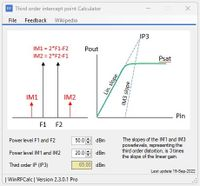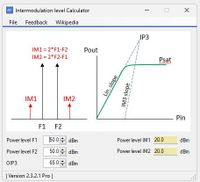# Third order intercept point (OIP3) calculator.

In the tool below you can see that the linear response of the amplification is limited by the saturated output power Psat. The effect of this limitation is the generation of inter-modulation products (IM1 and IM2)

The inter modulation analyzer deliverers the third order intercept point (IP3) which is a figure of merit of the product used.

In this calculator tool, the third order IP level in dBm, can interactively be calculated by changing the absolute power levels of the two main carriers and the two 3rd order inter-modulation products 2*F1-F2 and 2&F2-F1.In the calculator shown below, given the levels of carriers at F1, F2 and the 3OIP the power levels of the two IM products IM1 and IM2 are calculated.>100 RF Calculators
WinRFCalc, the best RF calculator toolbox for Windows